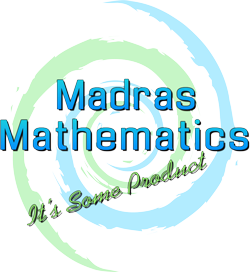# MRS SOARES1. Binomial Therom

SOLUTIONS

2. Complex Numbers 1(updated)

SOLUTIONS

3. Sequences & Series 1

SOLUTIONS

4. Sequences & Series 2

SOLUTIONS

5. Summations & Mathematical proofs

SOLUTIONS

6. Matrices 1

SOLUTIONS

7. Properties of functions(updated)

SOLUTIONS

8. Gaussian elimination

SOLUTIONS

9. Compex Numbers 2

SOLUTIONS

10. Matrices 2

SOLUTIONS

11. Partial Fractions

SOLUTIONS

12. Vectors

## COURSE NOTES

Advanced Higher course notes and examples from class, with filled in blanks.

Binomial Therom

Partial Fractions

Sequences and Series

Summation and Proof

Properties of Functions

Matrices and system of equations

Complex Numbers